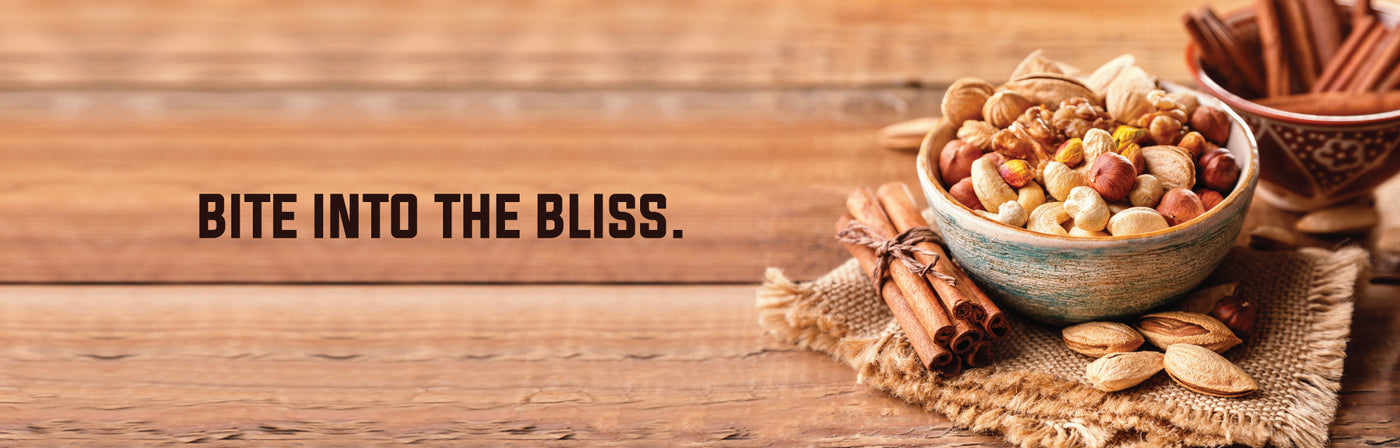Yummy SnacksSort by:
Rs. 250.00

Rs. 799.00

Rs. 299.00

From Rs. 169.00

Rs. 799.00

Rs. 199.00

Rs. 169.00

Rs. 199.00

Rs. 99.00

Rs. 399.00

Rs. 120.00

Rs. 160.00

Rs. 160.00

Rs. 120.00

Rs. 120.00

Rs. 120.00

From Rs. 169.00

Rs. 149.00

Rs. 169.00

Rs. 169.00

Rs. 199.00

Rs. 169.00

Rs. 149.00

From Rs. 199.00

Rs. 469.00

From Rs. 499.00

Rs. 169.00

Rs. 499.00

Rs. 299.00

Rs. 160.00

Rs. 79.00

Rs. 160.00

Rs. 399.00

Rs. 499.00

Rs. 699.00

Rs. 1,199.00

Rs. 499.00

Rs. 119.00

Rs. 169.00

Rs. 799.00

Rs. 399.00

From Rs. 289.00

Rs. 799.00

Rs. 1,499.00

Rs. 99.00

Rs. 99.00

Rs. 99.00

From Rs. 249.00

Sale

Unavailable

Sold Out